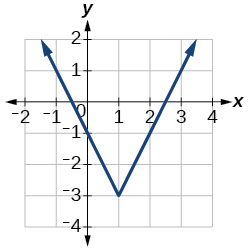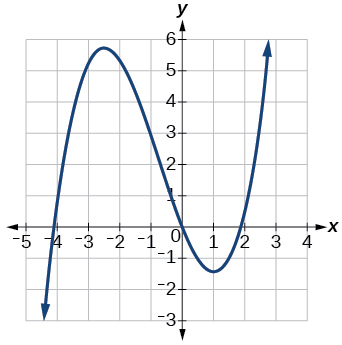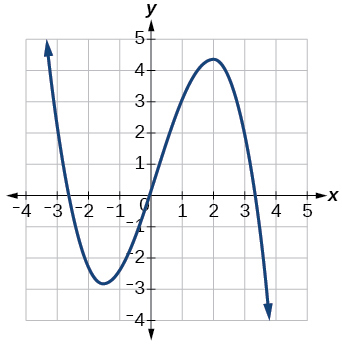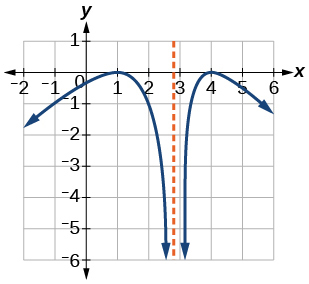# 3.3 Rates of change and behavior of graphs  (Page 5/15)

 Page 5 / 15

Access this online resource for additional instruction and practice with rates of change.

## Key equations

 Average rate of change $\frac{\Delta y}{\Delta x}=\frac{f\left({x}_{2}\right)-f\left({x}_{1}\right)}{{x}_{2}-{x}_{1}}$

## Key concepts

• A rate of change relates a change in an output quantity to a change in an input quantity. The average rate of change is determined using only the beginning and ending data. See [link] .
• Identifying points that mark the interval on a graph can be used to find the average rate of change. See [link] .
• Comparing pairs of input and output values in a table can also be used to find the average rate of change. See [link] .
• An average rate of change can also be computed by determining the function values at the endpoints of an interval described by a formula. See [link] and [link] .
• The average rate of change can sometimes be determined as an expression. See [link] .
• A function is increasing where its rate of change is positive and decreasing where its rate of change is negative. See [link] .
• A local maximum is where a function changes from increasing to decreasing and has an output value larger (more positive or less negative) than output values at neighboring input values.
• A local minimum is where the function changes from decreasing to increasing (as the input increases) and has an output value smaller (more negative or less positive) than output values at neighboring input values.
• Minima and maxima are also called extrema.
• We can find local extrema from a graph. See [link] and [link] .
• The highest and lowest points on a graph indicate the maxima and minima. See [link] .

## Verbal

Can the average rate of change of a function be constant?

Yes, the average rate of change of all linear functions is constant.

If a function $\text{\hspace{0.17em}}f\text{\hspace{0.17em}}$ is increasing on $\text{\hspace{0.17em}}\left(a,b\right)\text{\hspace{0.17em}}$ and decreasing on $\text{\hspace{0.17em}}\left(b,c\right),\text{\hspace{0.17em}}$ then what can be said about the local extremum of $\text{\hspace{0.17em}}f\text{\hspace{0.17em}}$ on $\text{\hspace{0.17em}}\left(a,c\right)?\text{\hspace{0.17em}}$

How are the absolute maximum and minimum similar to and different from the local extrema?

The absolute maximum and minimum relate to the entire graph, whereas the local extrema relate only to a specific region around an open interval.

How does the graph of the absolute value function compare to the graph of the quadratic function, $\text{\hspace{0.17em}}y={x}^{2},\text{\hspace{0.17em}}$ in terms of increasing and decreasing intervals?

## Algebraic

For the following exercises, find the average rate of change of each function on the interval specified for real numbers $\text{\hspace{0.17em}}b\text{\hspace{0.17em}}$ or $\text{\hspace{0.17em}}h$ in simplest form.

$f\left(x\right)=4{x}^{2}-7\text{\hspace{0.17em}}$ on

$4\left(b+1\right)$

$g\left(x\right)=2{x}^{2}-9\text{\hspace{0.17em}}$ on

$p\left(x\right)=3x+4\text{\hspace{0.17em}}$ on

3

$k\left(x\right)=4x-2\text{\hspace{0.17em}}$ on

$f\left(x\right)=2{x}^{2}+1\text{\hspace{0.17em}}$ on $\text{\hspace{0.17em}}\left[x,x+h\right]$

$4x+2h$

$g\left(x\right)=3{x}^{2}-2\text{\hspace{0.17em}}$ on $\text{\hspace{0.17em}}\left[x,x+h\right]$

$a\left(t\right)=\frac{1}{t+4}\text{\hspace{0.17em}}$ on $\text{\hspace{0.17em}}\left[9,9+h\right]$

$\frac{-1}{13\left(13+h\right)}$

$b\left(x\right)=\frac{1}{x+3}\text{\hspace{0.17em}}$ on $\text{\hspace{0.17em}}\left[1,1+h\right]$

$j\left(x\right)=3{x}^{3}\text{\hspace{0.17em}}$ on $\text{\hspace{0.17em}}\left[1,1+h\right]$

$3{h}^{2}+9h+9$

$r\left(t\right)=4{t}^{3}\text{\hspace{0.17em}}$ on $\text{\hspace{0.17em}}\left[2,2+h\right]$

$\frac{f\left(x+h\right)-f\left(x\right)}{h}\text{\hspace{0.17em}}$ given $\text{\hspace{0.17em}}f\left(x\right)=2{x}^{2}-3x\text{\hspace{0.17em}}$ on $\text{\hspace{0.17em}}\left[x,x+h\right]$

$4x+2h-3$

## Graphical

For the following exercises, consider the graph of $\text{\hspace{0.17em}}f\text{\hspace{0.17em}}$ shown in [link] .

Estimate the average rate of change from $\text{\hspace{0.17em}}x=1\text{\hspace{0.17em}}$ to $\text{\hspace{0.17em}}x=4.$

Estimate the average rate of change from $\text{\hspace{0.17em}}x=2\text{\hspace{0.17em}}$ to $\text{\hspace{0.17em}}x=5.$

$\frac{4}{3}$

For the following exercises, use the graph of each function to estimate the intervals on which the function is increasing or decreasing.increasing on $\text{\hspace{0.17em}}\left(-\infty ,-2.5\right)\cup \left(1,\infty \right),\text{\hspace{0.17em}}$ decreasing onincreasing on $\text{\hspace{0.17em}}\left(-\infty ,1\right)\cup \left(3,4\right),\text{\hspace{0.17em}}$ decreasing on $\text{\hspace{0.17em}}\left(1,3\right)\cup \left(4,\infty \right)$

For the following exercises, consider the graph shown in [link] .

#### Questions & Answers

show that the set of all natural number form semi group under the composition of addition
Nikhil Reply
what is the meaning
Dominic
explain and give four Example hyperbolic function
Lukman Reply
_3_2_1
felecia
⅗ ⅔½
felecia
_½+⅔-¾
felecia
The denominator of a certain fraction is 9 more than the numerator. If 6 is added to both terms of the fraction, the value of the fraction becomes 2/3. Find the original fraction. 2. The sum of the least and greatest of 3 consecutive integers is 60. What are the valu
SABAL Reply
1. x + 6 2 -------------- = _ x + 9 + 6 3 x + 6 3 ----------- x -- (cross multiply) x + 15 2 3(x + 6) = 2(x + 15) 3x + 18 = 2x + 30 (-2x from both) x + 18 = 30 (-18 from both) x = 12 Test: 12 + 6 18 2 -------------- = --- = --- 12 + 9 + 6 27 3
Pawel
2. (x) + (x + 2) = 60 2x + 2 = 60 2x = 58 x = 29 29, 30, & 31
Pawel
ok
Ifeanyi
on number 2 question How did you got 2x +2
Ifeanyi
combine like terms. x + x + 2 is same as 2x + 2
Pawel
x*x=2
felecia
2+2x=
felecia
×/×+9+6/1
Debbie
Q2 x+(x+2)+(x+4)=60 3x+6=60 3x+6-6=60-6 3x=54 3x/3=54/3 x=18 :. The numbers are 18,20 and 22
Naagmenkoma
Mark and Don are planning to sell each of their marble collections at a garage sale. If Don has 1 more than 3 times the number of marbles Mark has, how many does each boy have to sell if the total number of marbles is 113?
mariel Reply
Mark = x,. Don = 3x + 1 x + 3x + 1 = 113 4x = 112, x = 28 Mark = 28, Don = 85, 28 + 85 = 113
Pawel
how do I set up the problem?
Harshika Reply
what is a solution set?
Harshika
find the subring of gaussian integers?
Rofiqul
hello, I am happy to help!
Shirley Reply
please can go further on polynomials quadratic
Abdullahi
hi mam
Mark
I need quadratic equation link to Alpa Beta
Abdullahi Reply
find the value of 2x=32
Felix Reply
divide by 2 on each side of the equal sign to solve for x
corri
X=16
Michael
Want to review on complex number 1.What are complex number 2.How to solve complex number problems.
Beyan
yes i wantt to review
Mark
16
Makan
x=16
Makan
use the y -intercept and slope to sketch the graph of the equation y=6x
Only Reply
how do we prove the quadratic formular
Seidu Reply
please help me prove quadratic formula
Darius
hello, if you have a question about Algebra 2. I may be able to help. I am an Algebra 2 Teacher
Shirley Reply
thank you help me with how to prove the quadratic equation
Seidu
may God blessed u for that. Please I want u to help me in sets.
Opoku
what is math number
Tric Reply
4
Trista
x-2y+3z=-3 2x-y+z=7 -x+3y-z=6
Sidiki Reply
can you teacch how to solve that🙏
Mark
Solve for the first variable in one of the equations, then substitute the result into the other equation. Point For: (6111,4111,−411)(6111,4111,-411) Equation Form: x=6111,y=4111,z=−411x=6111,y=4111,z=-411
Brenna
(61/11,41/11,−4/11)
Brenna
x=61/11 y=41/11 z=−4/11 x=61/11 y=41/11 z=-4/11
Brenna
Need help solving this problem (2/7)^-2
Simone Reply
x+2y-z=7
Sidiki
what is the coefficient of -4×
Mehri Reply
-1
Shedrak

### Read also:

#### Get Jobilize Job Search Mobile App in your pocket Now!

Source:  OpenStax, College algebra. OpenStax CNX. Feb 06, 2015 Download for free at https://legacy.cnx.org/content/col11759/1.3
Google Play and the Google Play logo are trademarks of Google Inc.

Notification Switch

Would you like to follow the 'College algebra' conversation and receive update notifications?ByByBy John GabrieliBy Katie MontroseBy Sarah WarrenBy Anh DaoBy Janet ForresterBy Katy KeilersBy John GabrieliBy John GabrieliBy Yacoub JayoghliBy OpenStax